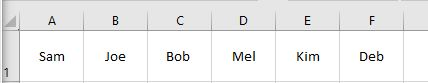# VBA Center Text – Cell Alignment (Horizontal & Vertical)

This tutorial will demonstrate how to use VBA to Center Text in Cells both Horizontally and Vertically.

We can use the Alignment group in the Home Ribbon in Excel to center text both horizontally and vertically in a cell. If we are writing a macro to format text, we can re-create this functionality using VBA Code.

## Center Text Horizontally

To Center Text horizontally in a single cell, we can use the following code:

``````Sub CenterText()
ActiveCell.HorizontalAlignment = xlCenter
End Sub``````

Alternatively, to center text horizontally in each cell of a selected range of cells, we can use the Selection object and do the following:

``````Sub CenterText()
Selection.HorizontalAlignment = xlCenter
End Sub``````

We can also change the alignment to right or left using the xlLeft and xlRight constants.

To right align the text in a cell, we can therefore use the following code:

``````Sub RightAlignText()
ActiveCell.HorizontalAlignment = xlRight
End Sub``````

## Center Text Vertically

Centering the text vertically is much the same as horizontally.

``````Sub CenterTextVertical()
ActiveCell.VerticalAlignment = xlCenter
End Sub``````

As is centering text vertically across a selection:

``````Sub CenterTextVertically()
Selection.VerticalAlignment = xlCenter
End Sub``````

We can also change the text to the Top or Bottom of a cell or selection using the xlTop or xlBottom constants.

``````Sub TopAlignVertically()
ActiveCell.VerticalAlignment = xlTop
End Sub``````

## Center Text Horizontally and Vertically at the Same Time

If we want to center the text both Horizontally and Vertically at the same time, there are a couple of ways we can do so.

``````Sub CenterBoth()
ActiveCell.HorizontalAlignment = xlCenter
ActiveCell.VerticalAlignment = xlCenter
End Sub``````

To cut down on repeating code, we can use a With and End With Statement.

``````Sub CenterBoth2()
With Selection
.HorizontalAlignment = xlCenter
.VerticalAlignment = xlCenter
End With
End Sub``````

The code above will apply to all the cells in Excel that are selected at the time.Using With and End With is very effective when we have a lot of formatting to do within the selection, such as merging cells or changing orientation.

``````Sub MergeAndCenter()
With Selection
.HorizontalAlignment = xlCenter
.VerticalAlignment = xlBottom
.Orientation = -36
.MergeCells = True
End With
End Sub``````

Stop searching for VBA code online. Learn more about AutoMacro - A VBA Code Builder that allows beginners to code procedures from scratch with minimal coding knowledge and with many time-saving features for all users!